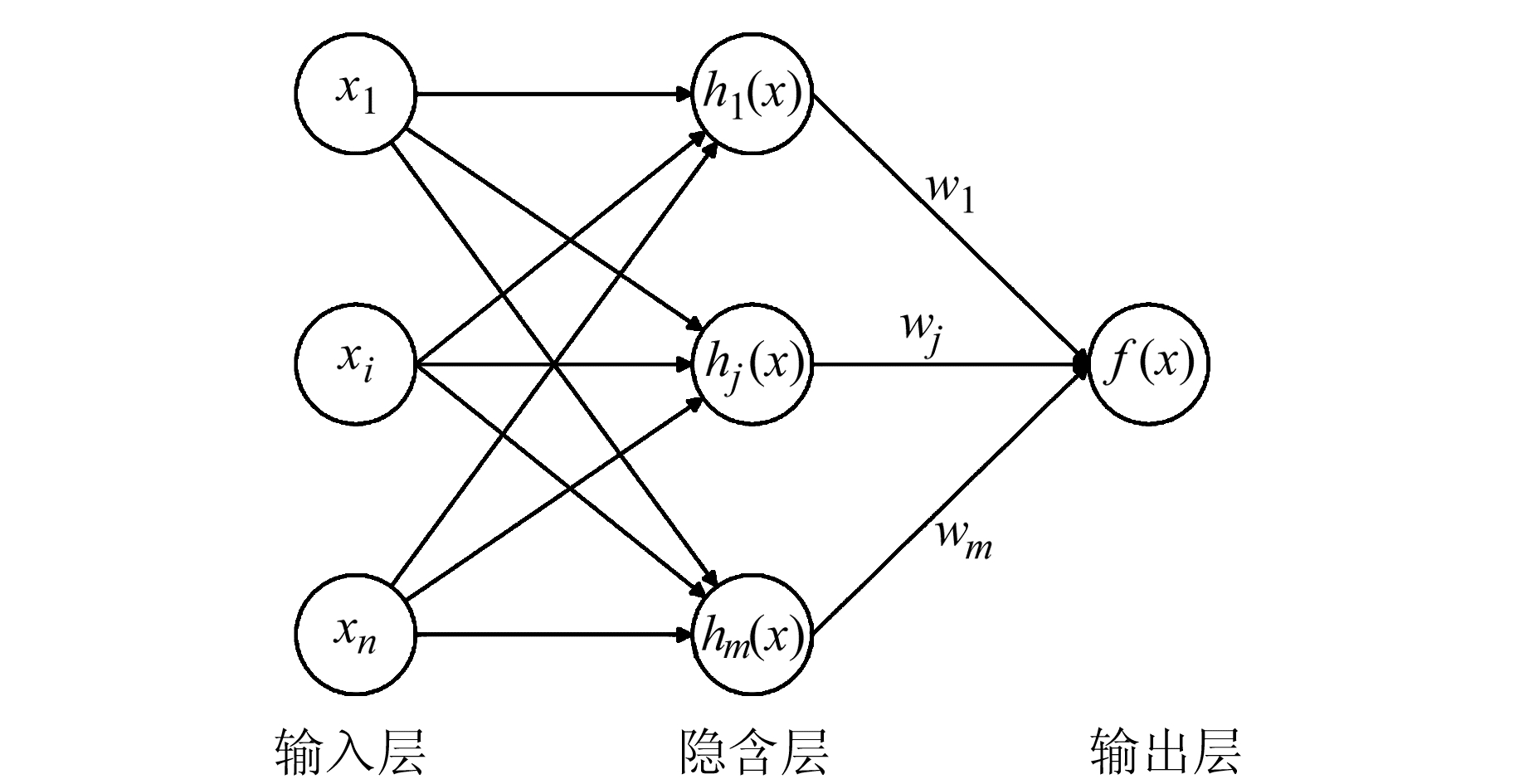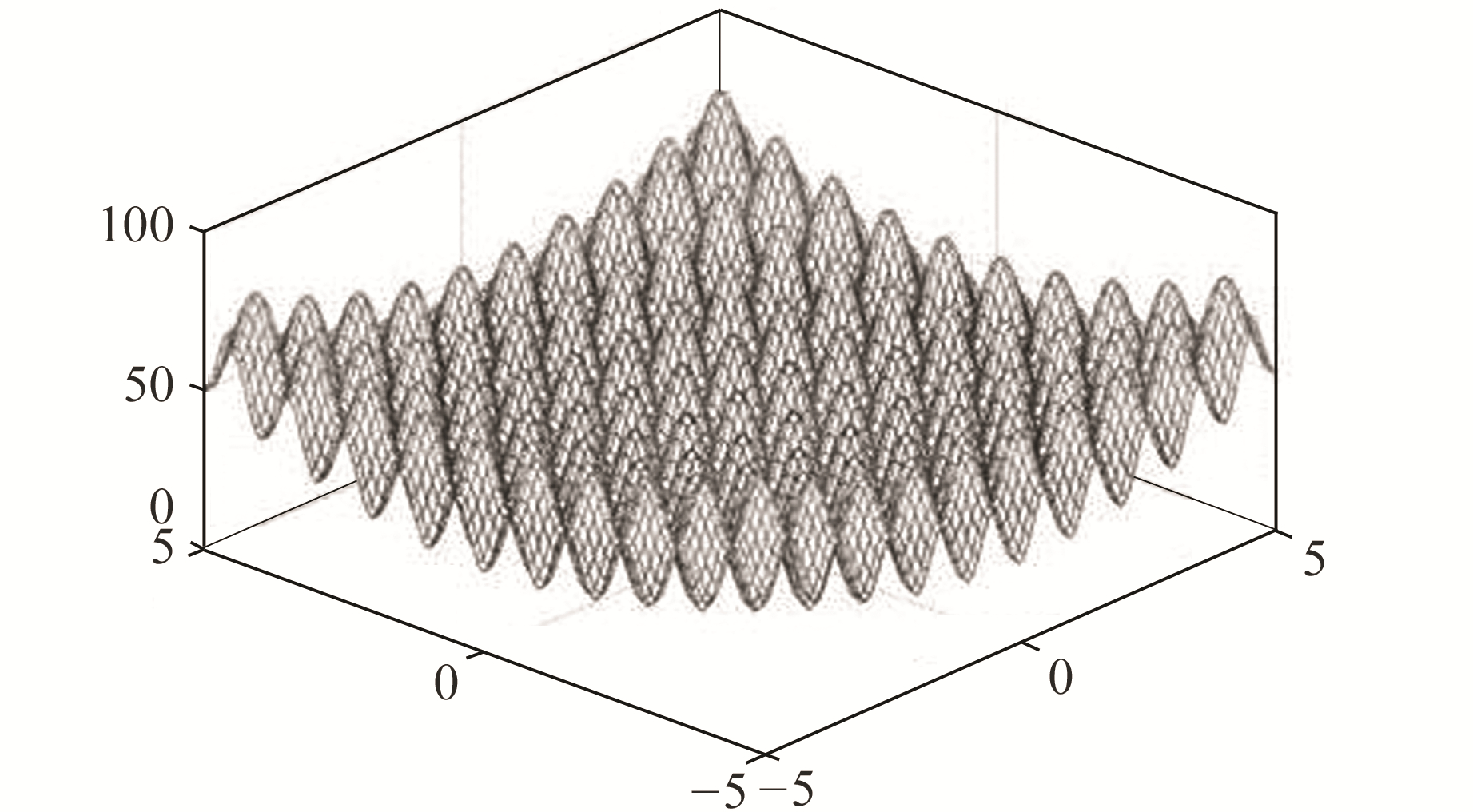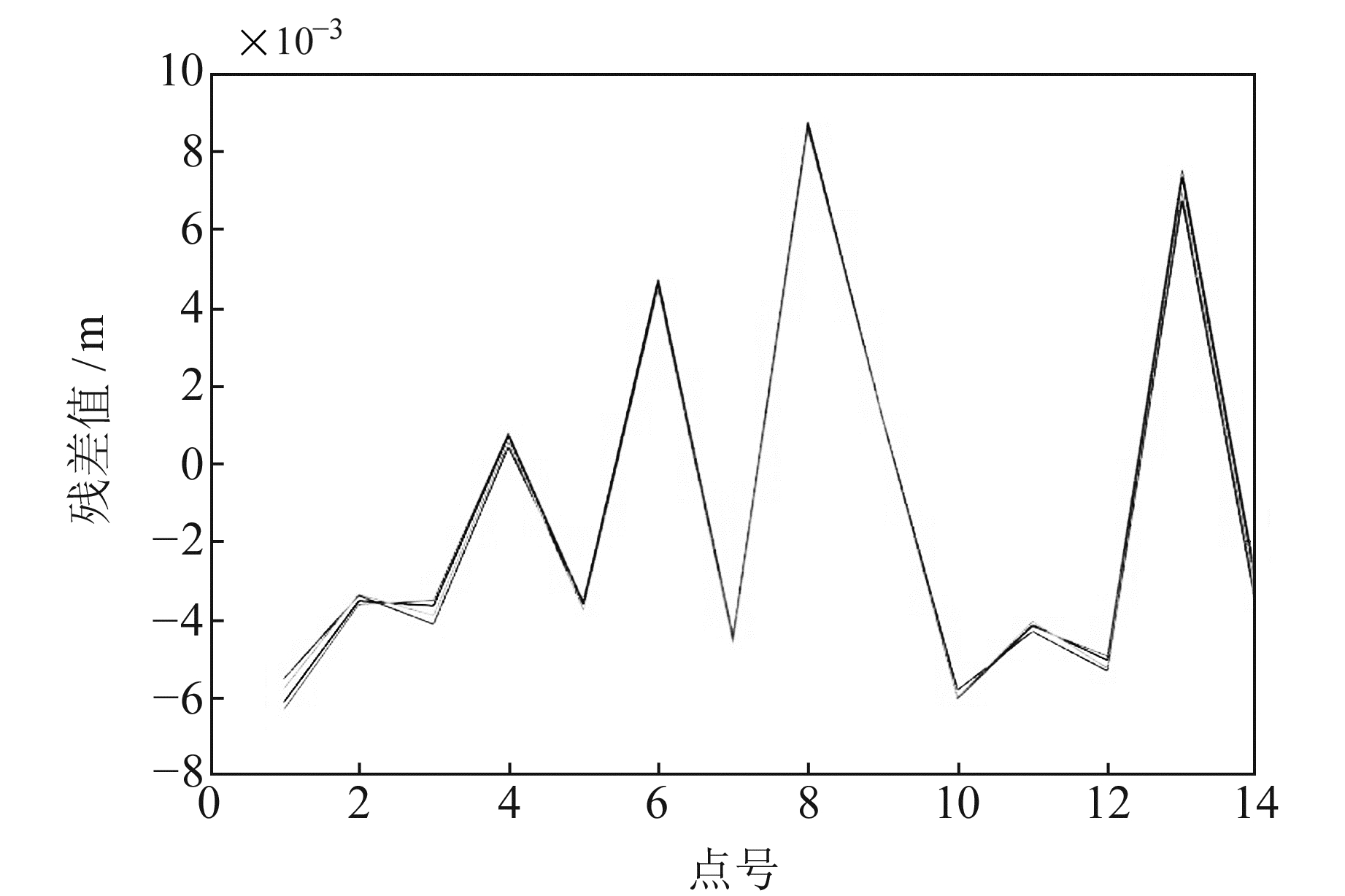﻿ 基于改进RBF神经网络的GNSS高程拟合文章快速检索 高级检索
 大地测量与地球动力学2020, Vol. 40Issue (3): 221-224, 241  DOI: 10.14075/j.jgg.2020.03.001### 引用本文YUAN Debao, ZHANG Jian, ZHAO Chuanwu, et al. GNSS Height Fitting Based on Improved RBF Neural Network[J]. Journal of Geodesy and Geodynamics, 2020, 40(3): 221-224, 241.### Foundation support

National Natural Science Foundation of China, No. 51474217.

### Corresponding author

ZHANG Jian, postgraduate, majors in GNSS data processing, E-mail: zjcumb@163.com.

### 第一作者简介

YUAN Debao, PhD, associate professor, majors in satellite navigation and positioning, E-mail: yuandb@cumtb.edu.cn.

### 文章历史

1. 中国矿业大学(北京)地球科学与测绘工程学院，北京市学院路丁11号，100083;
2. 中国地质大学(北京)信息工程学院，北京市学院路29号，100083

GNSS技术采用的坐标系统为地心坐标系统，其所测高程为大地高。由于工程实际中一般采用正常高，因此有必要将GNSS高程转换为正常高。

GNSS高程拟合基本原理为[1-3]

 $H=h+ε$ (1)

1 RBF神经网络高程拟合模型 1.1 RBF神经网络基本原理

RBF神经网络是一种3层前向网络，第1层为输入层，第2层为隐含层，第3层为输出层。其基本思想是通过隐含层将输入矢量转换到高纬度空间中，实现低维度空间内线性不可分问题在高维度空间内线性可分(图 1)。RBF神经网络基本学习算法流程如下：图 1 RBF神经网络拓扑结构 Fig. 1 Topological structure of RBF neural network

1) 输入训练样本，获取网络聚类中心ci，求取方差δi(i=1, 2, …, m)；

2) 基于径向基函数(高斯函数)求取隐含层输出值:

 $h_{j}=\sum\limits_{i=1}^{n} \exp \left(-\frac{\left\|x_{i}-c_{j}\right\|^{2}}{2 \delta_{j}^{2}}\right)$ (2)

3) 求取输出权值：

 $w_{j}=\exp \left(\frac{h}{c_{\max }}\left\|x_{p}-c_{i}\right\|^{2}\right)$ (3)

4) 求取网络输出值：

 $f(x)=\sum\limits_{i=1}^{m} h_{i}(x) w_{i}$ (4)
1.2 基于RBF神经网络GNSS高程拟合

1) GNSS拟合数据归一化处理；

2) 利用GNSS拟合数据建立RBF神经网络高程拟合模型；

3) 将检验点坐标(x, y)作为网络输入层，求取对应的高程异常值；同时，将外符合精度作为精度评价指标，确定模型拟合精度。

2 改进的RBF神经网络高程拟合模型

2.1 粒子群优化算法基本原理

 \begin{aligned} V_{i(d+1)}=& w \times v_{i d}+c_{1} r_{1}\left(P_{i d}-X_{i d}\right)+\\ & c_{2} r_{2}\left(P_{g d}-X_{i d}\right) \end{aligned} (5)
 $X_{i(d+1)}=X_{i d}+r \times v_{i d}$ (6)

2.2 改进的自适应权重粒子群优化算法

 $w=w_{\min }+\left(f-f_{\min }\right) \frac{w_{\max }-w_{\min }}{f_{\operatorname{avg}}-f_{\min }}$ (7)

 $w = {w_{\max }} - \left( {{w_{\max }} - {w_{\min }}} \right)\frac{1}{{1 + {{\rm{e}}^{a - bt}}}}$ (8)

2.3 仿真实验分析图 2 多峰值函数 Fig. 2 Multi-peak function表 1 粒子群算法寻优情况 Tab. 1 Particle swarm optimization
2.4 基于改进RBF神经网络的GNSS高程拟合

1) 导入GNSS拟合数据，平面坐标(x, y)作为输入向量，高程异常值作为输出向量；

2) 利用MATLAB mapminmax函数将数据归一化处理；

4) 将测试数据代入训练好的RBF神经网络模型，获取拟合值；同时，将外符合精度作为精度评价指标，确定模型拟合精度。

3 实验分析图 3 10次实验拟合残差值 Fig. 3 Fitting residual values of 10 experiments表 2 各模型的外符合精度 Tab. 2 External coincidence accuracy of each model
4 结语

  蒲伦, 唐诗华, 张紫萍, 等. 基于蚁群算法的多面函数在GPS高程拟合中的应用[J]. 大地测量与地球动力学, 2019, 39(1): 31-35 (Pu Lun, Tang Shihua, Zhang Ziping, et al. Application of Multi-Quadric Function Based on Ant Colony Algorithm in GPS Elevation Fitting[J]. Journal of Geodesy and Geodynamics, 2019, 39(1): 31-35) (0)  刘建, 王琪洁, 王小辉, 等. 基于遗传算法的RBF神经网络在GPS高程拟合中的应用[J]. 测绘科学, 2013, 38(2): 143-145 (Liu Jian, Wang Qijie, Wang Xiaohui, et al. Application of RBF Neural Network to GPS Elevation Fitting Based on Genetic Algorithm[J]. Science of Surveying and Mapping, 2013, 38(2): 143-145) (0)  陈智伟, 张兴福, 胡波, 等. 利用RBF实现线状工程中GNSS高程转换的精度分析[J]. 测绘通报, 2018(12): 79-82 (Chen Zhiwei, Zhang Xingfu, Hu Bo, et al. Using RBF to Analyze the Accuracy of GNSS Height Transformation in Linear Engineering[J]. Bulletin of Surveying and Mapping, 2018(12): 79-82) (0)  方苏阳, 赵勇. GPS高程拟合方法对比研究分析[J]. 全球定位系统, 2018, 43(4): 110-116 (Fang Suyang, Zhao Yong. Experimental Research on GPS Height Fitting Method[J]. GNSS World of China, 2018, 43(4): 110-116) (0)  张海燕, 李秀海, 韩冰. 基于三次样条函数的长线路GNSS高程拟合研究[J]. 测绘工程, 2018, 27(7): 15-17 (Zhang Haiyan, Li Xiuhai, Han Bing. Research on GNSS Height Fitting for Long Line Area Based on Cubic Spline Function[J]. Engineering of Surveying and Mapping, 2018, 27(7): 15-17) (0)  Tao Y Q, Mao G X, Zhou X Z. Solution for GNSS Height Anomaly Fitting of Mining Area Based on Robust TLS[J]. Acta Geodaetica et Geophysica, 2018, 53(2): 295-307 DOI:10.1007/s40328-018-0217-0 (0)  杨帆, 王小兵, 谢洋洋, 等. 灰色神经网络在GPS高程拟合中的应用[J]. 导航定位学报, 2017, 5(3): 90-93 (Yang Fan, Wang Xiaobing, Xie Yangyang, et al. Application of Grey Neural Network in GPS Height Fitting[J]. Journal of Navigation and Positioning, 2017, 5(3): 90-93 DOI:10.3969/j.issn.2095-4999.2017.03.018) (0)  魏宗海. 几种改进的BP神经网络在GPS高程拟合中的应用比较[J]. 全球定位系统, 2016, 41(5): 99-103 (Wei Zonghai. Comparison of Several Improved BP Neural Network in GPS Height Fitting[J]. GNSS World of China, 2016, 41(5): 99-103) (0)  刘斌, 郭际明, 史俊波, 等. 利用EGM2008模型与地形改正进行GPS高程拟合[J]. 武汉大学学报:信息科学版, 2016, 41(4): 554-558 (Liu Bin, Guo Jiming, Shi Junbo, et al. A GPS Height Fitting Method Based on the EGM2008 Model and Terrain Correction[J]. Geomatics and Information Science of Wuhan University, 2016, 41(4): 554-558) (0)  石长伟, 何晴晴, 陈长坤. RBF神经网络在区域性GPS高程拟合中的应用与精度分析[J]. 测绘与空间地理信息, 2018, 41(11): 94-96 (Shi Changwei, He Qingqing, Chen Changkun. Application and Accuracy Analysis of RBF Neural Network in Regional GPS Height Fitting[J]. Geomatics and Spatial Information Technology, 2018, 41(11): 94-96 DOI:10.3969/j.issn.1672-5867.2018.11.027) (0)  彭中波, 高阳. BP神经网络在水下地形高程拟合的应用[J]. 重庆交通大学学报:自然科学版, 2018, 37(11): 64-68 (Peng Zhongbo, Gao Yang. Application of BP Neural Network in Elevation Fitting of Underwater Terrain[J]. Journal of Chongqing Jiaotong University: Natural Science, 2018, 37(11): 64-68) (0)  束蝉方, 李斐, 李明峰. 基于RBF神经网络的GPS/水准高程异常拟合[J]. 地球物理学进展, 2011, 26(3): 819-823 (Shu Chanfang, Li Fei, Li Mingfeng. GPS/Leveling Quasi-Geoid Fitting Based on RBF Neural Networks[J]. Progress in Geophysics, 2011, 26(3): 819-823 DOI:10.3969/j.issn.1004-2903.2011.03.005) (0)  高宁, 王晓静, 王静燕. 基于RBF神经网络的矿区GPS高程转换研究[J]. 煤炭技术, 2015, 34(10): 110-112 (Gao Ning, Wang Xiaojing, Wang Jingyan. Research on Transformation of GPS Height in Mining Area Based on Radial Basis Function Neural Network[J]. Coal Technology, 2015, 34(10): 110-112) (0)  黄洋, 鲁海燕, 许凯波, 等. 基于S型函数的自适应粒子群优化算法[J]. 计算机科学, 2019, 46(1): 245-250 (Huang Yang, Lu Haiyan, Xu Kaibo, et al. S-shaped Function Based Adaptive Particle Swarm Optimization Algorithm[J]. Computer Science, 2019, 46(1): 245-250) (0)
GNSS Height Fitting Based on Improved RBF Neural Network
YUAN Debao1ZHANG Jian1ZHAO Chuanwu1     DU Shigao2     Peng Jinying1
1. College of Geoscience and Surveying Engineering, China University of Mining and Technology, D11 Xueyuan Road, Beijing 100083, China;
2. School of Information Engineering, China University of Geosciences, 29 Xueyuan Road, Beijing100083, China
Abstract: In the traditional RBF neural network model in the GNSS height fitting, the fitting accuracy is relatively low, the stability is relatively poor, and the correlation factors need to be set artificially in advance. This paper adopts the improved adaptive weight particle swarm optimization algorithm and MATLAB RBF newrb. The network function newrb combines to realize the automatic optimization of the number of hidden nodes and SPREAD in the RBF neural network function model, and improve the accuracy and stability of the algorithm in GNSS height fitting. Through the example analysis, the method has high fitting precision and can reach mm precision. Compared with the traditional quadratic polynomial model, the accuracy is improved by 17% and the stability is good. It has important reference value for accurately solving GNSS height anomaly.
Key words: GNSS; height fitting; improved particle swarm optimization; RBF neural network; MATLAB